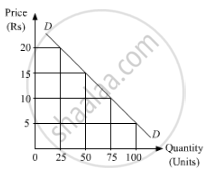Share

# Do You Agree with the Following Statement? Give Reason State and Explain the Law of Demand. - Economics

ConceptDemand Curve and Its Slope

#### Question

Do you agree with the following statement? Give reason

State and explain the law of demand.

#### Solution

The law of demand focuses on the basic relationship between the price of the good and quantity demanded of the good.

According to this law, other things being constant (ceteris paribus), a consumer’s demand shares an inverse relationship with the price of a good and vice versa. In other words, if the income, price of related goods and taste and preferences of the consumer remain unchanged, then the demand for the good moves in the opposite direction of its price.

The law of demand is based on the following assumptions:

1. Size of the population remains the same.
2. Income of the consumer remains unchanged.
Prices of related goods remain unchanged.
3. Consumer’s tastes and preferences remain unchanged.
Government’s policies remain unchanged.
4. There is no change in the expectations about the future.

The law of demand can be explained with the help of the following demand schedule:

 Price of Commodity (X)(Rs) Quantity Demanded of X(Units) 5 100 10 75 15 50 20 25

The schedule shows that as the price of commodity X increases from Rs 10 to Rs 15, the quantity demanded for X falls from 75 units to 50 units. Thus, there is a negative relationship between demand and price.Let us plot the above schedule on a graph. On the x-axis, we represent the quantity demanded; and on the y-axis, we represent the price of the good. Joining the different combinations of price and quantity demanded, we get a curve DD. This curve is the demand curve showing the inverse relationship between the price and the quantity demanded.

Is there an error in this question or solution?

#### APPEARS IN

Micheal Vaz Solution for Micheal Vaz Class 12 Economics (2019 to Current)
Chapter 3: Demand Analysis
Exercise | Q: 1 | Page no. 24
Solution Do You Agree with the Following Statement? Give Reason State and Explain the Law of Demand. Concept: Demand Curve and Its Slope.
S